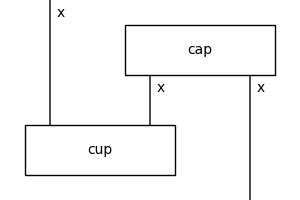# diagramize#

discopy.drawing.legacy.diagramize(dom, cod, boxes, factory=None)[source]#

Define a diagram using the syntax for Python functions.

Parameters
Returns

decorator – Decorator which turns a function into a diagram.

Return type

function

Example

```>>> from discopy.rigid import Ty, Cup, Cap
>>> x = Ty('x')
>>> cup, cap = Cup(x, x.r), Cap(x.r, x)
>>> @diagramize(dom=x, cod=x, boxes=[cup, cap])
... def snake(left):
...     middle, right = cap(offset=1)
...     cup(left, middle)
...     return right
>>> snake.draw(
...     figsize=(3, 3), path='docs/_static/drawing/diagramize.png')
```